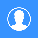مدرس اون لاين

## 12022012Impulse and Momentum

Impulse and Momentum

Momentum

* The momentum of a body is equal to its mass multiplied by its velocity.

Momentum is measured in N s. Note that momentum is a vector quantity, in other words the direction is important.
Impulse

The impulse of a force (also measured in N s) is equal to the change in momentum of a body which a force causes. This is also equal to the magnitude of the force multiplied by the length of time the force is applied.

* Impulse = change in momentum = force × time

Conservation of Momentum

When there is a collision between two objects, Newton's Third Law states that the force on one of the bodies is equal and opposite to the force on the other body.

Therefore, if no other forces act on the bodies (in the direction of collision), then the total momentum of the two bodies will be unchanged. Hence the total momentum before collision in a particular direction = total momentum after in a particular direction.

This can be used to solve problems involving colliding spheres (see also: restitution).
Example

We have the following scenario (a ball of mass 3kg is moving to the right with velocity 3m/s and a ball of mass 1kg is moving to the left with velocity 2m/s):Suppose we are told that after the collision, the ball of mass 1kg moves away with velocity 2m/s, then we can use the principle of conservation of momentum to determine the velocity of the other ball after the collision.Initial momentum = 3.3 - 2.1 = 7 [the minus sign is important: it is there because the velocity of the 1kg body is in the opposite direction to the velocity of the 3kg body].

Final momentum = 2 - 3x

Hence 7 = 2 - 3x (since momentum is conserved)

x = -5/3

Which means that my arrow was pointing in the wrong direction (because of the minus sign), hence the velocity of the 3kg body after the collision is 5/3 ms-1 to the right.

http://goo.gl/Ng1YL

## تعاليقmerci beaucoup
privacy_tip صلاحيات هذا المنتدى:
لاتستطيع الرد على المواضيع في هذا المنتدى